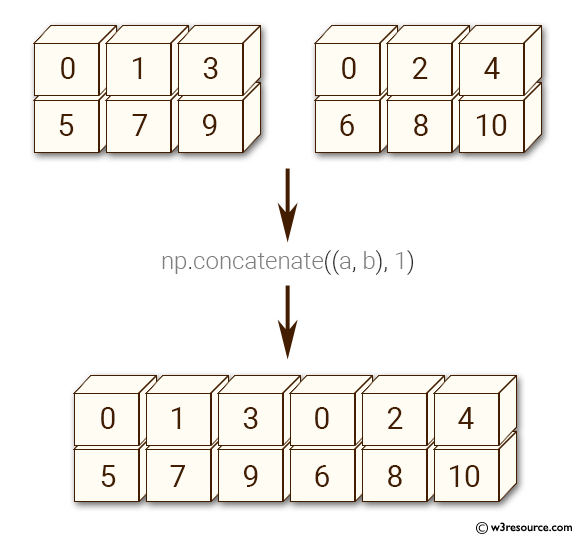﻿ NumPy: Concatenate two 2-dimensional arrays - w3resource# NumPy: Concatenate two 2-dimensional arrays

## NumPy: Array Object Exercise-58 with Solution

Write a NumPy program to concatenate two 2-dimensional arrays.
Sample arrays: ([[0, 1, 3], [5, 7, 9]], [[0, 2, 4], [6, 8, 10]]

Pictorial Presentation:Sample Solution:-

Python Code:

``````import numpy as np
a = np.array([[0, 1, 3], [5, 7, 9]])
b = np.array([[0, 2, 4], [6, 8, 10]])
c = np.concatenate((a, b), 1)
print(c)
```
```

Sample Output:

```[[ 0  1  3  0  2  4]
[ 5  7  9  6  8 10]]
```

Python Code Editor:

Have another way to solve this solution? Contribute your code (and comments) through Disqus.

What is the difficulty level of this exercise?

Test your Python skills with w3resource's quiz

﻿

## Python: Tips of the Day

Set comprehension:

```>>> m = {x ** 2 for x in range(5)}
>>> m
{0, 1, 4, 9, 16}
```